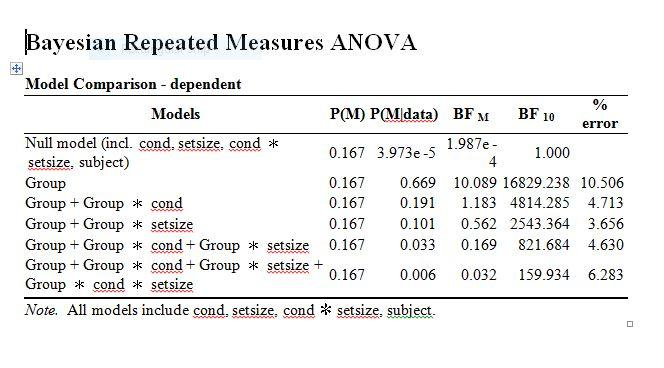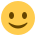#### Howdy, Stranger!

It looks like you're new here. If you want to get involved, click one of these buttons!

Supported by

# Interpreting Bayesian RM ANOVA output

Hi E.J.,

I was wondering if you could advise whether my analysis below is correct. I ran Bayesian repeated measure ANOVA on JASP and have attached the output to this post. I'm interested in knowing whether (a) group and cond interact, (b) group and setsize interact; and (c) 3-way interaction: cond x setsize x group. Here are my findings based on the output:

(a) group x cond: 821.684/2543.364 = 0.32. I concluded there is evidence for the null

(b) group x setsize: 821.684/4814.285 = 0.17. I concluded also there is evidence for the null

(c) 3-way interaction: 821.684/159.934 = 5.13. Again, there is evidence against the null.***

***The above results ( a and b) are in line with the ANOVA except for (c), that is, the 3-way interaction. The ANOVA showed a non-significant 3-way interaction.

Please could you kindly advise if my analysis and interpretation above are correct? Thank you so much in advance.

Toc• Hi Toc,

I suspect that your analysis is not correct. But I'm having trouble understanding why you made the specific comparisons that you have. Could you explain the logic behind what you've done?

As it happens, I just posted a blog about interpreting Bayesian Repeated Measures output:

Cheers!
Sebastiaan

• At least (c) seems the other way around
E.J.

• Hi Sebastiaan,

Thanks for your comment and sharing the link. I find it useful. Ok, here it goes. I have two separate studies. Study 1 has two factors: cond and setsize. Study 2 also has two factors: cond and set size. I have ran separate Bayesian repeated measures ANOVA for both studies and everything seems fine. Therefore, I want to compare the two studies to see if performance is better in study 1 compared to study 2 and if it is, whether the advantage is mediated by cond and set size. In fact, the analysis is analogous to Mixed-factorial ANOVA, where study 1 and 2 (designated as group in the output) is between subject factor and cond and setsize as within subject factors. As you can see from the output, there is overwhelming evidence for H0 for the effect of group, indicating differences in performance between the two studies. So I'm interested in knowing if:

a) there is interaction between group and cond. That is whether performance the effect of condition is higher in study 1 than study 2
b) there is interaction between group and setsize. That is whether performance the effect of setsize is greater in study 1 than study 2
c) whether there is a 3-way interaction: group x cond x setsize.

Following the analysis and applying the transitive formula, I compared:

a) the model containing group, cond, and setsize (5th row in the output) with the model containing group and setsize (3rd row) = 821.684/2543.364 = 0.32. Therefore, I concluded there is evidence against the interaction (i.e., Group x cond)

b) the model containing group, cond, and setsize (5th row in the output) with the model containing group and cond (2nd row) = 821.684/4814.285 = 0.172. Also, I concluded there is evidence against the interaction (i.e., Group x setsize)

c) for the 3-way interaction (i.e., group x cond x setize), I compared the model on row 6 with the model on row 5 = 821.684/159.934 = 5.13. Thus, I concluded there is evidence for the interaction as H0 is supported. However, as E.J. said, it should be the other way round, that will then be 159.934/821.684 = 0.19. In that case, it is fair to conclude there is evidence against the 3-way interaction.

I hope I have made this clearer now. My question then is, is the analysis and interpretation correct?

Toc

• Hi,

If I understand correctly, you have marked a whole bunch of model terms as 'Is Nuisance'. Since none of the terms are really a nuisance (?), I assume you did this to reduce the number of models in the table so that direct model comparison becomes feasible. Is that right?

I wouldn't do that. What I would do is unmark all your terms as nuisance. This will give you a big, messy model table. But you can then apply the Baws Factor logic from the post that I linked to above to arrive at the evidence for specific terms. Does that make sense? It takes a bit of manual labor, but it's not difficult.

Cheers,
Sebastiaan

• Thanks Sebastiaan. Yes, you are right. I added cond, set size and cond x setsize as nuisance variable to allow for direct model comparison. I have read your post on Baws Factor, though it takes time but its very easy and straight forward.

Once again, thanks for your support and all suggestions. Much appreciated.

Cheers,

Toc

• Hi Sebastiaan, given that the Baws factor is derived from P(M|data), how would one go ahead and report the result since I noticed most people report Bayes factor? So for instance, in a paper where I'm reporting three separate experiments, Experiment 1 and 2 have two factors each. The interpretation was based on information from BF10 (transitive formula applied), hence in the write-up I mentioned for instance, there is a compelling evidence against the null model for the interaction between factor 1 and factor 2 (Bayes factor = 23.37 or BF10 = 23.37). Then I come to my third experiment where I used Baws factor, will I still be reporting Bayes factor or Baws factor? Obviously the reviewer might ask, why the sudden change in terminology. Also, how do I reference Baws factor in my paper?

Thank you once again for all your suggestions.

Cheers, Toc

• Hi Toc,

I think Sebastiaan was not beging serious when he proposed "Baws" as a name. You might just want to describe what models you were averaging over to compute the inclusion probabilities. Oh boy, we do need that manual and some decent help files...

Cheers,
E.J.

• Ok, thanks E.J. for your response. I'm sure the manual will save some lives when it is out.

Cheers,

Toc